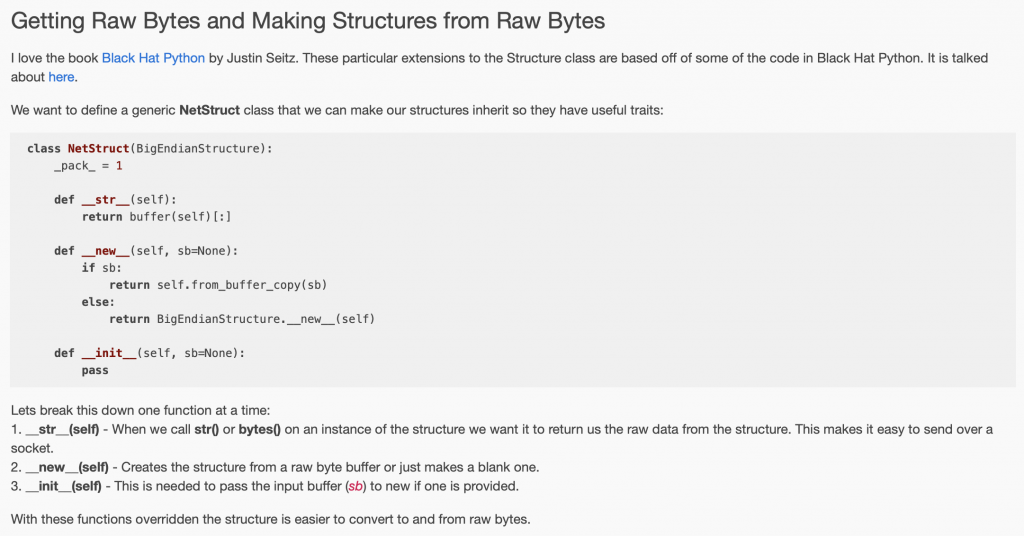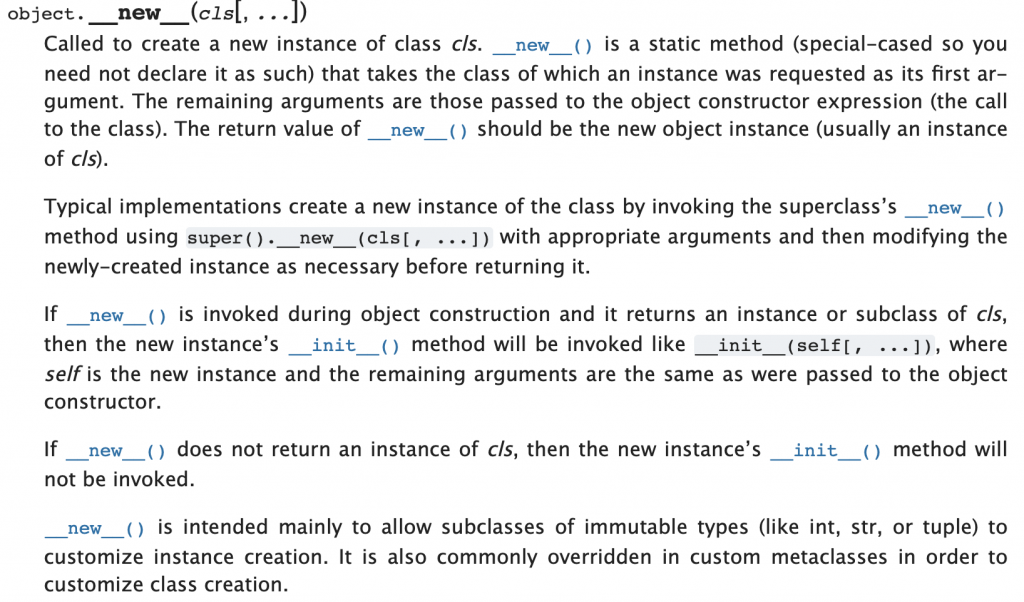# Summary

A discussion of overriding __new__ and __init__ to simplify the creation Python cstruct.Structure objects.

# Creating a new cytpes.BigEndianStructure

Here is a simple example of a 2-byte structure using the ctypes.BigEndianStructure class.

In this example I:

• Derive a new class called MyStruct from the BigEndianStructure
• Declare three fields called first (4-bits), second (4-bits) and third (8-bits).
• Create a new object of MyStruct type called “a”
• Set the values of the three fields
• Print it out.
```import ctypes

class MyStruct(ctypes.BigEndianStructure):
_pack_ = 1
_fields_ = [    ("first",ctypes.c_uint8,4),
("second",ctypes.c_uint8,4),
("third",ctypes.c_uint8,8),
]

# Create a blank MyStruct
a = MyStruct()
a.first  = 0xa
a.second = 0xb
a.third  = 0xcd
print(bytes(a))```

• Notice that it is BigEndian (which is good since that is what I declared)
• 0xA = 4-bits, 0-xB=4-bit so 0xAB is the first byte.
```(venv) \$ python ex-struct.py
b'\xab\xcd'```

You can also create a new structure by calling the class method “from_buffer_copy” with parameter of bytes type.

```# Create a MyStruct initialized to Hex ABCD
b = MyStruct.from_buffer_copy(b"\xab\xcd")
print(bytes(b))```

When you run this, you get the same result.

```(venv) \$ python ex-struct.py
b'\xab\xcd'```

What I was really hoping to be able to do is create a new structure from an array of bytes like this:

```# Create a new MyStruct from an Array of bytes ... this is gonna crash
c = MyStruct(b"\x0abb\xcd")
print(bytes(c))```

But that crashes.

```(venv) \$ python ex-struct.py
b'\xab\xcd\x00\x00'
b'\nbb\xcd'
Traceback (most recent call last):
File "ex-struct.py", line 24, in <module>
c = MyStruct(b"\x0abb\xcd")
TypeError: an integer is required (got type bytes)
(venv) \$```

And for some reason this took me a really long time to figure out.  You probably say to yourself, “Im not surprised it took him so long to figure out.  He is programming in Python so he probably isn’t very smart anyway”

# Overriding __new__ & __init__

While working to understand, I ran into the article “A better way to work with raw data types in Python” which I found interesting.  Here is a screen shot of a bit of the code.OK.  So lets add the dunder init and dunder new methods to my class.

```class MyStruct1(ctypes.BigEndianStructure):
_pack_ = 1
_fields_ = [    ("first",ctypes.c_uint8,4),
("second",ctypes.c_uint8,4),
("third",ctypes.c_uint8,8),
]

def __new__(self,sb=None):
if(sb):
return self.from_buffer_copy(sb)
else:
return ctypes.BigEndianStructure.__new__(self)

def __init__(self,sb=None):
pass

print("Next case")

c = MyStruct1()
c.first  = 0xa
c.second = 0xb
c.third  = 0xcd
print(bytes(c))

d = MyStruct1(b'\xab\xcd')
print(bytes(d))```

Now when I run it, things are good.

```(venv) \$ python ex-struct.py

Next case
b'\xab\xcd'
b'\xab\xcd'```

# Why do I need the __init__?

So, why do I need the dunder init that doesn’t actually do anything? Presumably if I was a real python programmer I would have already known the answer.  But I’m not, so I didn’t.

The first question I had is what does the Python keyword “pass” do?  The answer is nothing.  It is a nop or void if you prefer and is there just to make the program legal as a function has to have some function.

Then onto the Python documentation where I found that if you have an __new__ method that returns an object of the subtype Python will automatically call the __init__ function.# A Conundrum

```def __str__(self):